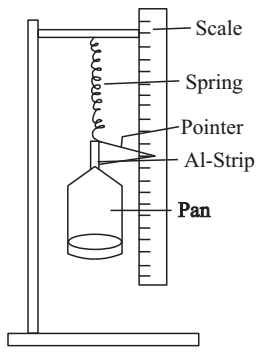# Helical Spring Experiment

Experiment: To measure extensions in the length of a helical spring with increasing load.

It follows from Hooke’s law that the gravitational force of load M suspended in a spring and extension produced in it by the load are proportional to each other.

Mg ∝ l

⇒ Mg = μl

μ = mg/l

Here μ is the force in newton required to produce unit extension and is called spring constant of the spring.

If you plot a graph between extension produced l (on y - axis) and load suspended Mg (on x-axis) then

μ = change in weight / change in l

= 1 / slope of graph

This equation gives the value of μ in the SI unit (Nm–1).

Material Required

The spring, a pan which can be suspended below the spring, weight box, half-metre scale, laboratory stand, light aluminium strip with a pointer

### How To Set Up

Attach the scale in the laboratory stand in a vertical position. On the same stand suspend the spring. Suspend a light aluminium strip below it at which is stuck a light paper pointer. At the lower end of the strip suspend the pan. When weights are added in the pan and spring extends, tip of the pointer moves down on the scale, without touching it. Position of the tip of the pointer can be read on the scale.### How To Perform Experiment

1. Note the zero reading of pointer on the scale with no weights in the pan. Add a suitable weight, M, in the pan and note the new reading on the scale. Difference of the two readings gives extension, I, of the spring due to the weight M.

2. Gradually increase in steps the weights in the pan and note the position of pointer for each load.

3. After an appropriate maximum load is reached, reduce the weights in same steps. Again note the position of pointer for each load. If the spring has not been permanently strained by your maximum load, the pointer will return to its previous position for each load. There can be some observational error. Hence find the mean of the two readings and then extension for each load.

4. Plot a graph between extension l (on y-axis) and load M (on x-axis). Draw the best straight line through the points plotted and the origin, which is also an observation - zero extension for zero load.

5. Find the slope of the graph and then the constant μ.

μ = change in M / change in l

= 1 / slope of graph

### Sources of Error

1. If pointer positions for equal loads for load decreasing are lower than those for load increasing, then a permanent extension of spring has occurred by the maximum load applied. At that load, Hook’s law breaks down.
2. There can be friction between the pointer and the scale if they touch each other and their contact is not light enough. Then, for a given load, the pointer will come to rest in several positions.